Examples

Chapter 12 Class 10 Surface Areas and Volumes
Serial order wise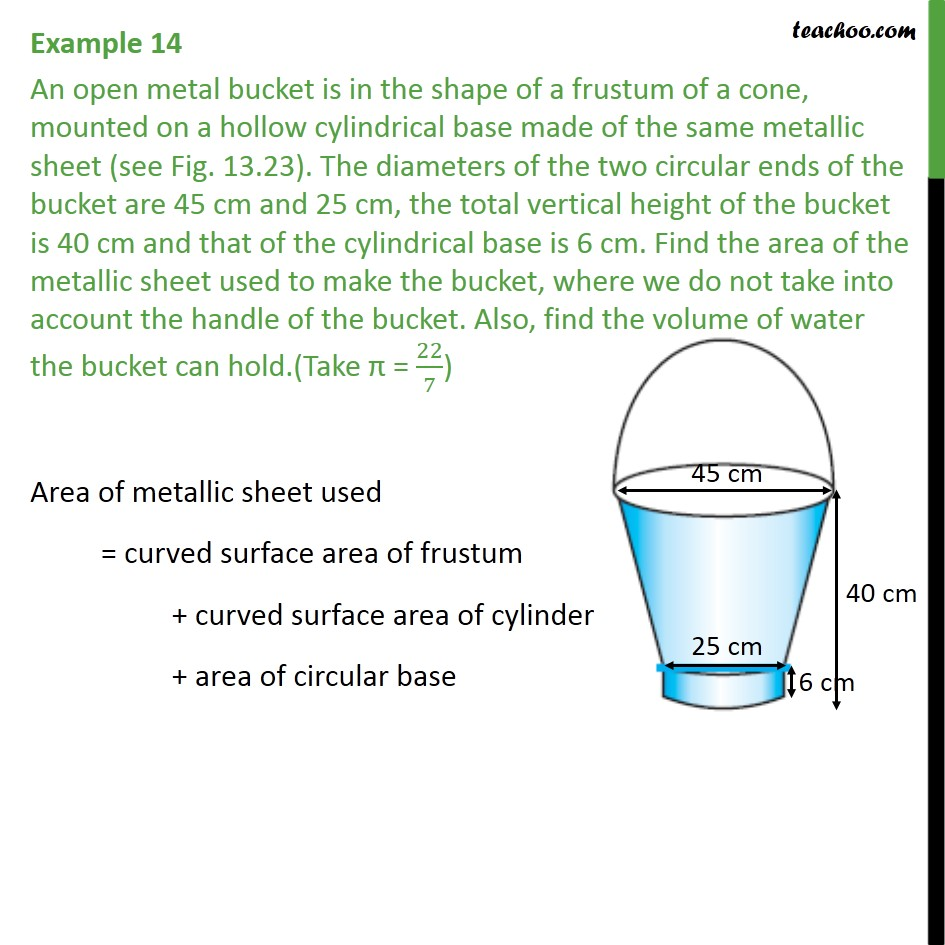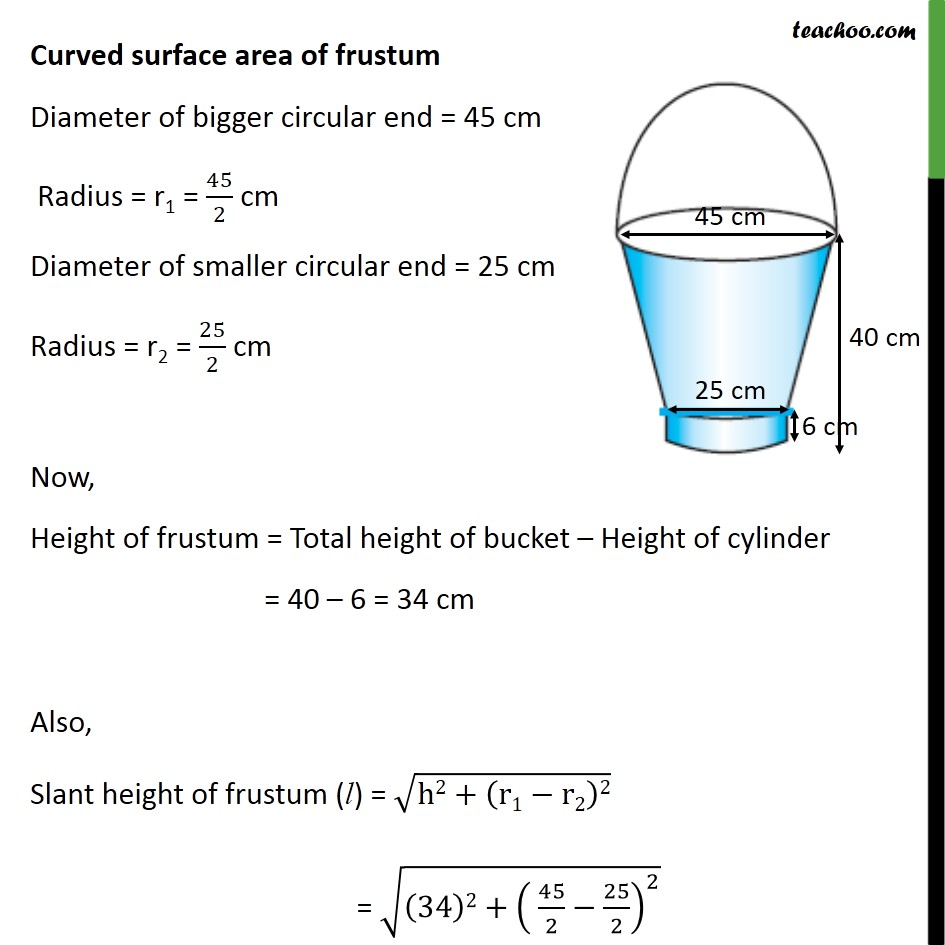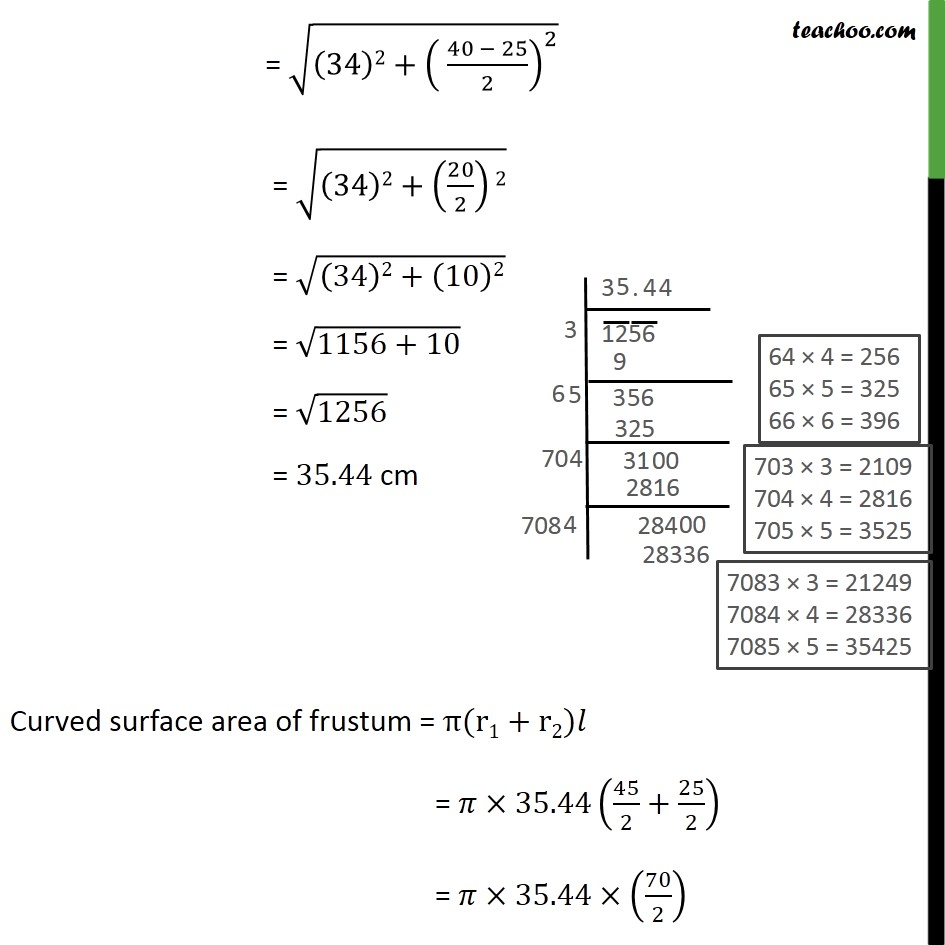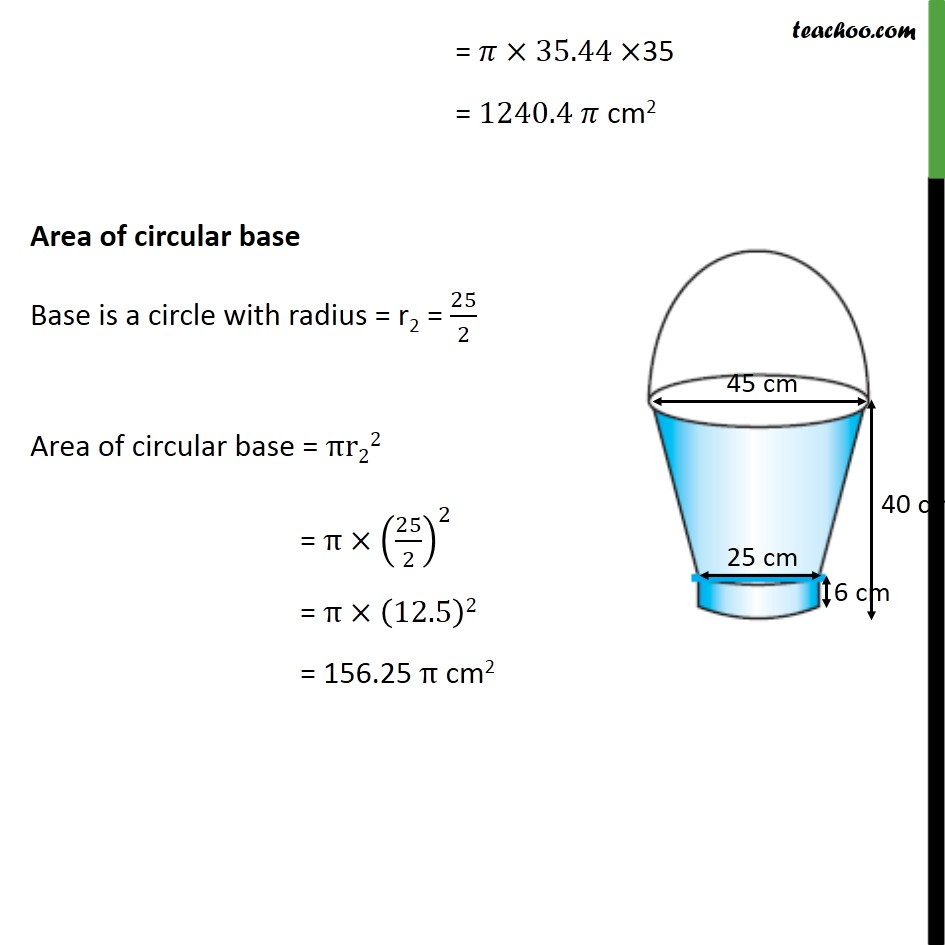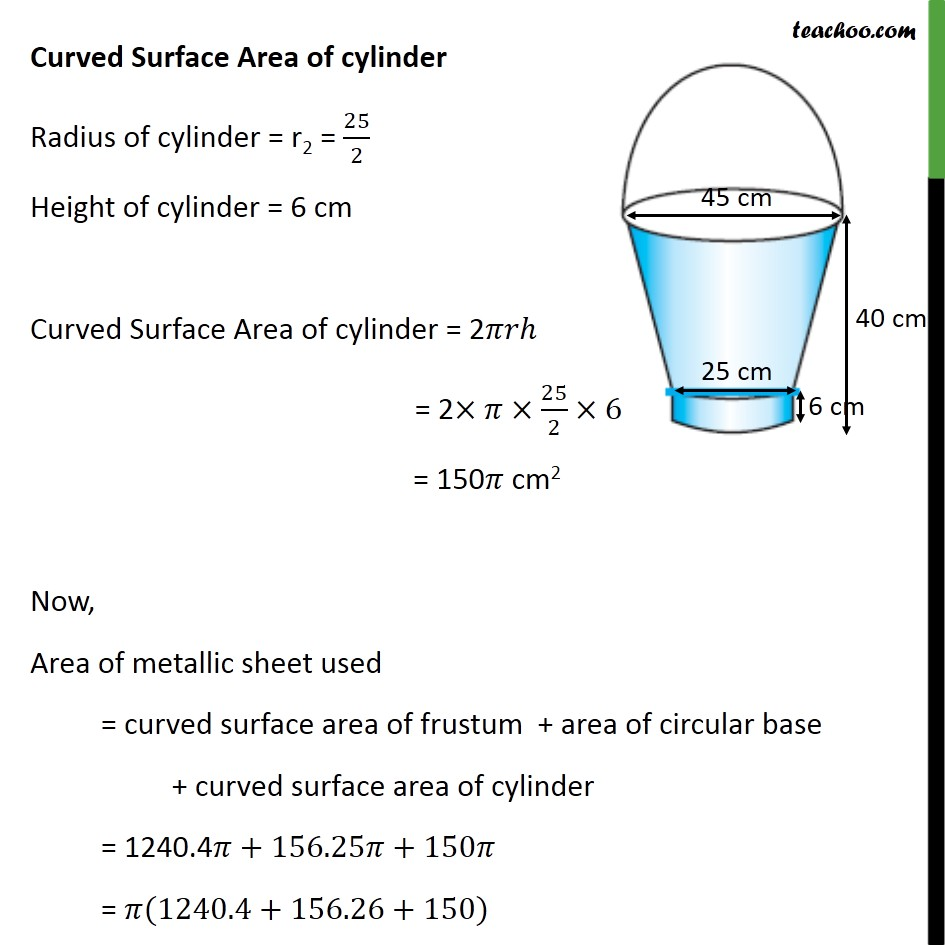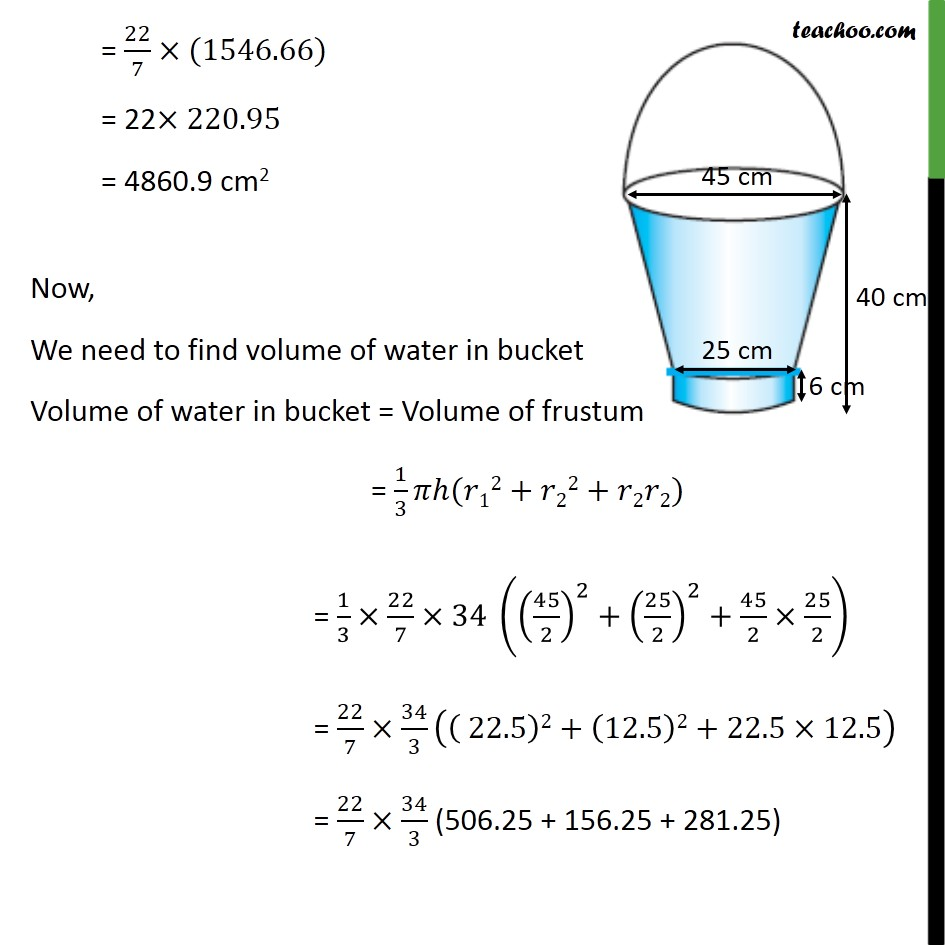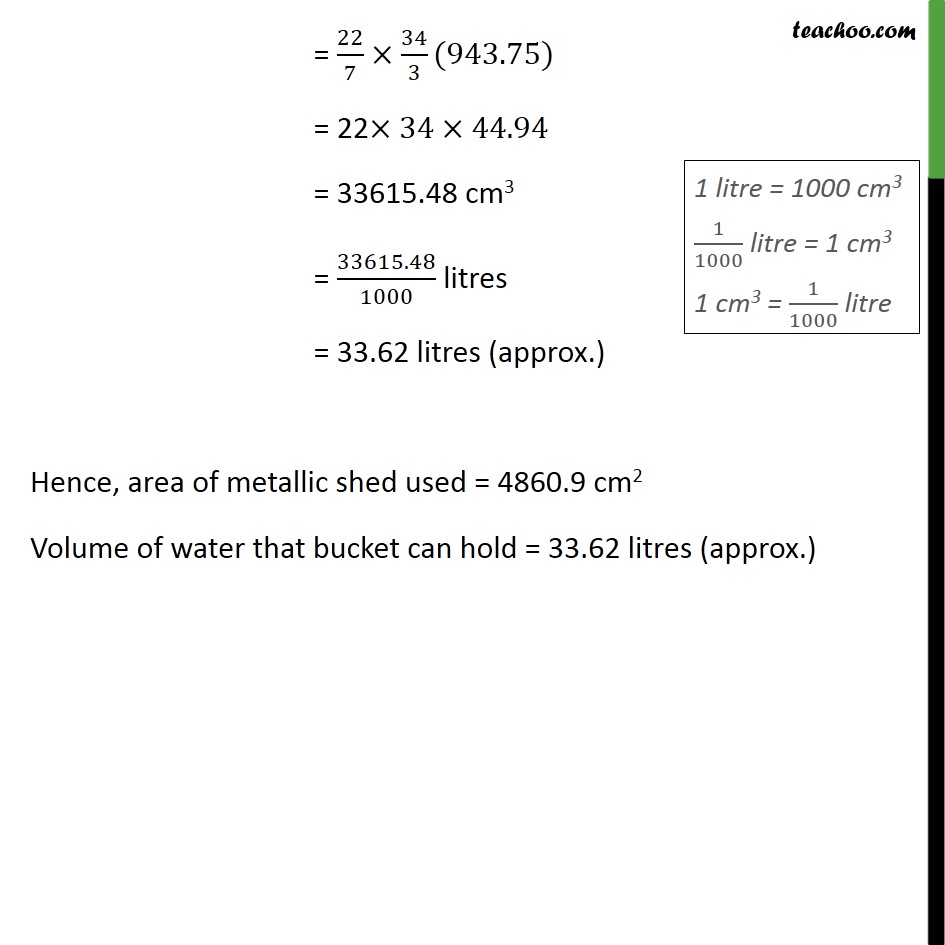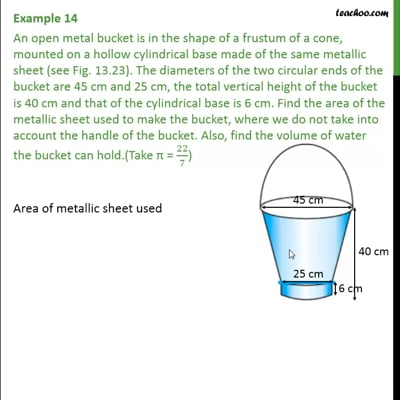This video is only available for Teachoo black users

Learn in your speed, with individual attention - Teachoo Maths 1-on-1 Class

### Transcript

Question 7 An open metal bucket is in the shape of a frustum of a cone, mounted on a hollow cylindrical base made of the same metallic sheet (see Fig. 13.23). The diameters of the two circular ends of the bucket are 45 cm and 25 cm, the total vertical height of the bucket is 40 cm and that of the cylindrical base is 6 cm. Find the area of the metallic sheet used to make the bucket, where we do not take into account the handle of the bucket. Also, find the volume of water the bucket can hold.(Take = 22/7) Area of metallic sheet used = curved surface area of frustum + curved surface area of cylinder + area of circular base Curved surface area of frustum Diameter of bigger circular end = 45 cm Radius = r1 = 45/2 cm Diameter of smaller circular end = 25 cm Radius = r2 = 25/2 cm Now, Height of frustum = Total height of bucket Height of cylinder = 40 6 = 34 cm Also, Slant height of frustum (l) = (h2+(r1 r2)2) = ((34)2+( 45/2 25/2)^2 ) = ((34)2+( (40 25)/2)^2 ) = ((34)2+(20/2)2) = ((34)2+(10)2) = (1156+10) = 1256 = 35.44 cm Curved surface area of frustum = (r1+r2) = 35.44(45/2+25/2) = 35.44 (70/2) = 35.44 35 = 1240.4 cm2 Area of circular base Base is a circle with radius = r2 = 25/2 Area of circular base = r22 = (25/2)^2 = (12.5)2 = 156.25 cm2 Curved Surface Area of cylinder Radius of cylinder = r2 = 25/2 Height of cylinder = 6 cm Curved Surface Area of cylinder = 2 = 2 25/2 6 = 150 cm2 Now, Area of metallic sheet used = curved surface area of frustum + area of circular base + curved surface area of cylinder = 1240.4 +156.25 +150 = (1240.4+156.26+150) = 22/7 (1546.66) = 22 220.95 = 4860.9 cm2 Now, We need to find volume of water in bucket Volume of water in bucket = Volume of frustum = 1/3 ( 12+ 22+ 2 2) = 1/3 22/7 34 ((45/2)^2+(25/2)^2+45/2 25/2) = 22/7 34/3 (( 22.5)2+(12.5)2+22.5 12.5) = 22/7 34/3 (506.25 + 156.25 + 281.25) = 22/7 34/3(943.75) = 22 34 44.94 = 33615.48 cm3 = 33615.48/1000 litres = 33.62 litres (approx.) Hence, area of metallic shed used = 4860.9 cm2 Volume of water that bucket can hold = 33.62 litres (approx.)